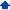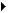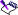### Performing regression analysis

Regression analysis answers the question “To what extent can one or more independent variables explain and predict a dependent variable?” By performing regression analysis, you can create a regression analysis table showing how sets of independent variables affect a certain set of dependent variables. For example, you can estimate how the number of hours worked affects production, or how advertising expenditures affect sales.

The relationship between dependent and independent variables is a linear estimate. With one independent variable, regression analysis plots a line of best fit, also called a regression line, through a scatter plot of each independent-dependent value pair.

Because regressions assume a linear relationship, the results will be more accurate if the data closely matches a linear model. To help you determine accuracy of results, the regression table includes two standard error estimates.

#### To perform regression analysis1 Click ToolsNumeric toolsRegression.
 2 Click the Range pickerin the Dependent box, and select the column of dependent data.
 3 Click the Range pickerin the Independent box, and select the column of independent data.
 4 Click the Range pickerin the Output box, and select the upper-left cell of the output cells where you want to write the regression information.
 If you want to force the y-intercept to zero, enable the Zero option in the Y intercept area.• The data cells you compare in regression analysis must have an equal number of rows.
 • Regression tables are not automatically updated.